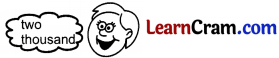# DAV Class 3 Maths Chapter 1 Worksheet 3 Solutions

The DAV Class 3 Maths Book Solutions and DAV Class 3 Maths Chapter 1 Worksheet 3 Solutions of Numbers upto 9999 offer comprehensive answers to textbook questions.

## DAV Class 3 Maths Ch 1 WS 3 Solutions

Question 1.

(a) 2000
Solution:
2000 = Two thousand two thousand(b) 4321
Solution:
4321 = Four thousand three hundred twenty one.

(c) 7701
Solution:
7701 = Seven thousand seven hundred one.

(d) 2508
Solution:
2508 = Two thousand five hundred eight.(e) 8006
Solution:
8006 = Eight thousand six.

(f) 9998
Solution:
9998 = Nine thousand nine hundred ninety eight.

(g) 7256
Solution:
7256 = Seven thousand two hundred fifty six.

(h) 6066
Solution:
6066 = Six thousand sixty six.Question 2.
Write the number names for the following:

(a) 7000
Solution:
7000 = Seven thousand.

(b) 7312
Solution:
7312 = Seven thousand three hundred twelve

(c) 6806
Solution:
6806 = Six thousand eight hundred six.

(d) 4509
Solution:
4509 = Four thousand five hundred nine.

(e) 9009
Solution:
9009 = Nine thousand nine.

(f) 9993
Solution:
9993 = Nine thousand nine hundred ninety three.

(g) 4356
Solution:
4356 = Four thousand three hundred fifty six.

(h) 2020
Solution:
2020 = Two thousand twenty.Question 3.
Write the numerals for the following:

(a) Four thousand five hundred nine.
Solution:
4509

(b) Three thousand six.
Solution:
3006

(c) Five thousand nine hundred fifty.
Solution:
5950

(d) Four thousand eighty nine.
Solution:
4089

(e) Four thousand eight hundred five.
Solution:
4805

(f) Two thousand twenty.
Solution:
2020

(g) Six thousand six hundred sixty six.
Solution:
6666

(h) Nine thousand nine.
Solution:
9009### DAV Class 3 Maths Chapter 1 Worksheet 3 NotesNumbers Number Names 4000 Four thousand 6500 Six thousand five hundred 7341 Seven thousand three hundred forty one.